2018-07-05 17:08:50 u010960265 阅读数 846
• ###### 老镇讲堂-Swift动画编程指南

掌握Swift动画编程

25854 人正在学习 去看看 曾伟（老镇）

## 2. UIView动画

① frame动画
② transform动画

#### 2.1 设置UIView动画的两种语法形式

begin — commit

//偏移动画
[UIView beginAnimations:@"move" context:nil];
[UIView setAnimationDuration:2];
[UIView setAnimationDelegate:self];
imageContainView.frame = CGRectMake(80, 80, 200, 200);
[label1 setBackgroundColor:[UIColor yellowColor]];
[label1 setTextColor:[UIColor redColor]];
[UIView commitAnimations];


animations block

//缩放动画
view.transform = CGAffineTransformIdentity;
[UIView animateWithDuration:1.0f animations:^{
view.transform = CGAffineTransformMakeScale(2.0f, 2.0f);
}];


#### 2.2 设置属性形变动画的两种类型

UIView的 CGAffineTransform 类型属性：animatedView.transform

CGAffineTransform transform = CGAffineTransformScale(imageContainView.transform, 1.2, 1.2);
[UIView beginAnimations: @"scale"context: nil];
[UIView setAnimationDuration: 2];
[UIView setAnimationDelegate: self];
[imageView setTransform: transform];
[UIView commitAnimations];


CALayer的CATransform3D 类型属性：animaView.layer.transform

imageView.layer.transform =  CATransform3DIdentity;
[UIView animateWithDuration:1.0f animations:^{
imageView.layer.transform = CATransform3DMakeScale(2.0, 2.0, 1.0);
}];


#### 2.3 与动画相关的属性

###### 2.3.1 UIView与动画相关的属性–与CGAffineTransform对应

@property(nonatomic) CGRect            frame;
@property(nonatomic) CGRect            bounds;      // default bounds is zero origin, frame size. animatable
@property(nonatomic) CGPoint           center;      // center is center of frame. animatable
@property(nonatomic) CGAffineTransform transform;   // default is CGAffineTransformIdentity. animatable
@property(nonatomic) CGFloat           contentScaleFactor NS_AVAILABLE_IOS(4_0);

@property(nonatomic,getter=isMultipleTouchEnabled) BOOL multipleTouchEnabled __TVOS_PROHIBITED;   // default is NO
@property(nonatomic,getter=isExclusiveTouch) BOOL       exclusiveTouch __TVOS_PROHIBITED;         // default is NO


(1) 当涉及一些frame, bounds, center的改变或是形变的时候可以用 transform 来取代 frame。
(2) 一般在实际开发中都是平移，旋转，缩放组合使用。

######2.3.2 CALayer与动画相关的属性–与CATransform3D对应

//宽度和高度
@property CGRect bounds;

//位置(默认指中点，具体由anchorPoint决定)
@property CGPoint position;

//锚点(x,y的范围都是0-1)，决定了position的含义
@property CGPoint anchorPoint;

//背景颜色(CGColorRef类型)
@property CGColorRef backgroundColor;

//形变属性
@property CATransform3D transform;

//边框颜色(CGColorRef类型)
@property CGColorRef  borderColor;

//边框宽度
@property CGFloat borderWidth;

//圆角半径

//内容(比如设置为图片CGImageRef)
@property(retain) id contents;


#### 2.4 管理二维形变和三维形变的封装类：CGAffineTransform与CATransform3D

###### 2.4.1 CGAffineTransform操作API

CGAffineTransform结构体定义


struct CGAffineTransform {
CGFloat a, b, c, d;
CGFloat tx, ty;
};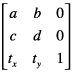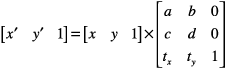a表示x水平方向的缩放，tx表示x水平方向的偏移
d表示y垂直方向的缩放，ty表示y垂直方向的偏移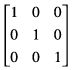#### CGAffineTransform操作API

//还原
CGAffineTransformIdentity

//位移仿射  ---- 理解为平移 (CGFloat tx,CGFloat ty)
CGAffineTransformMakeTranslation
CGAffineTransformTranslate
//旋转仿射 ---- 理解为旋转 (CGFloat angle)
CGAffineTransformMakeRotation
CGAffineTransformRotate
//缩放仿射  --- 理解缩放大小 (CGFloat sx, CGFloat sy)
CGAffineTransformMakeScale
CGAffineTransformScale


CGAffineTransform操作的数学本质

CGPoint转换公式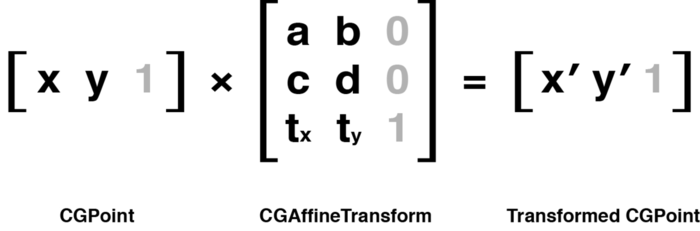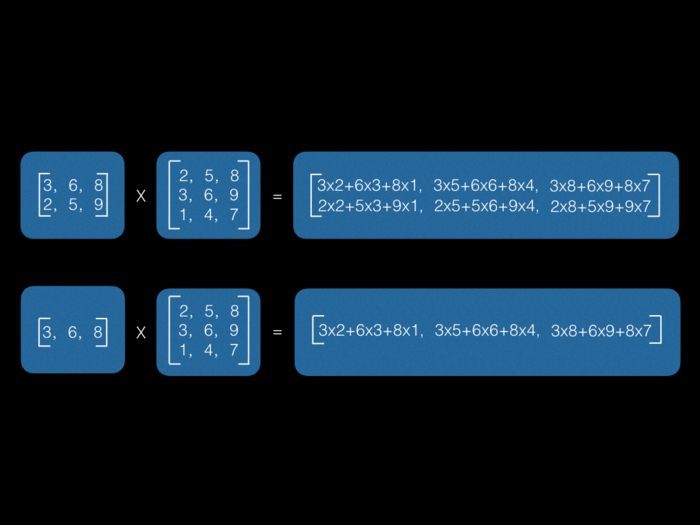###### 2.4.2 CATransform3D操作API
//还原
CATransform3DIdentity

//位移3D仿射  ==> (CGFloat tx, CGFloat ty, CGFloat tz)
CATransform3DMakeTranslation
CATransform3DTranslation
//旋转3D仿射 ==> (CGFloat angle, CGFloat x, CGFloat y, CGFloat z)
CATransform3DMakeRotation
CATransform3DRotation
//缩放3D仿射 ==>  (CGFloat angle, CGFloat x, CGFloat y, CGFloat z)
CATransform3DMakeScale
CATransform3DScale
//叠加3D仿射效果
CATransform3DConcat
//仿射基础3D方法，可以直接做效果叠加
CGAffineTransformMake (sx,shx,shy,sy,tx,ty)
//检查是否有做过仿射3D效果  == ((CATransform3D t))
CATransform3DIsIdentity(transform)
//检查2个3D仿射效果是否相同
CATransform3DEqualToTransform(transform1,transform2)
//3D仿射效果反转（反效果，比如原来扩大，就变成缩小）
CATransform3DInvert(transform)

###### 2.4.3 CATransform3D与CGAffineTransform相互转换API
//将一个CGAffinrTransform转化为CATransform3D
CATransform3D CATransform3DMakeAffineTransform (CGAffineTransform m);
//判断一个CATransform3D是否可以转换为CAAffineTransformbool
CATransform3DIsAffine (CATransform3D t);
//将CATransform3D转换为CGAffineTransform
CGAffineTransform CATransform3DGetAffineTransform (CATransform3D t);


#### 2.5 “组合动画” 与 CGAffineTransformConcat

###### 2.5.1 连接设置多个属性组合成一个动画

alertView.transform = CGAffineTransformMakeScale(.25, .25);

###### 2.5.2 利用CGAffineTransformConcat设置组合动画

CGAffineTransform viewTransform = CGAffineTransformConcat(CGAffineTransformMakeScale(.25, .25), CGAffineTransformMakeTranslation(0, 600));


CGAffineTransformConcat 组合多种形变动画小例子

- (void)viewDidAppear: (BOOL)animated {
///  初始化动画开始前label的位置
CGFloat offset = label1.frame.size.height * 0.5;

label1.transform = CGAffineTransformConcat(
CGAffineTransformMakeScale(0, 0),
CGAffineTransformTranslate(0, -offset)
);
label1.alpha = 0;
[UIView animateWithDuration: 3. animations: ^ {
///  还原label1的变换状态并形变和偏移label2
label1.transform = CGAffineTransformIdentifier;
label1.transform = CGAffineTransformConcat(
CGAffineTransformMakeScale(0, 0),
CGAffineTransformTranslate(0, offset)
);
label1.alpha = 1;
label2.alpha = 0;
}];
}


CGAffineTransformConcat的数学本质是将括号内代表的若干变换的系数矩阵进行相乘。

CGAffineTransform transform = CGAffineTransformIdentity;
transform = CGAffineTransformScale(transform, 0.5f, 0.5f);
transform = CGAffineTransformRotate(transform, 30.0f/180.0f*M_PI);
transform = CGAffineTransformTranslate(transform, 200.0f, 0.0f);
layerView.layer.affineTransform = transform;


#### 2.6 动画后将属性还原

view.transform = CGAffineTransformIdentity;
view.layer.transform = CATransform3DIdentity;


#### 2.7 注意点: transform对frame的影响

Changes to this property can be animated. However, if the transform
property contains a non-identity transform, the value of the frame
property is undefined and should not be modified. In that case, you
can reposition the view using the center property and adjust the size

## 3. CAAnimation核心动画

CAAnimation——所有动画对象的父类

#### 3.1 设置动画的一种语法形式

/**
*  抖动效果
*/
-(void)shakeAnimation{
CAKeyframeAnimation *anima = [CAKeyframeAnimation animationWithKeyPath:@"transform.rotation"];//在这里@"transform.rotation"==@"transform.rotation.z"
NSValue *value1 = [NSNumber numberWithFloat:-M_PI/180*4];
NSValue *value2 = [NSNumber numberWithFloat:M_PI/180*4];
NSValue *value3 = [NSNumber numberWithFloat:-M_PI/180*4];
anima.values = @[value1,value2,value3];
anima.repeatCount = MAXFLOAT;

}


#### 3.2 CAAnimation继承结构

CAAnimation{
CAPropertyAnimation{
CABasicAnimation{
CASpringAnimation
}
CAKeyframeAnimation
}
CATransition
CAAnimationGroup
}


#### 3.3 CAAnimation类的属性

• *duration：动画的持续时间
• *repeatCount：重复次数，无限循环可以设置HUGE_VALF或者MAXFLOAT
• *repeatDuration：重复时间
• removedOnCompletion：默认为YES，代表动画执行完毕后就从图层上移除，图形会恢复到动画执行前的状态。如果想让图层保持显示动画执行后的状态，那就设置为NO，不过还要设置fillMode为kCAFillModeForwards
• *fillMode：决定当前对象在非active时间段的行为。比如动画开始之前或者动画结束之后
• *beginTime：可以用来设置动画延迟执行时间，若想延迟2s，就设置为
• CACurrentMediaTime()+2，CACurrentMediaTime()为图层的当前时间
• timingFunction：速度控制函数，控制动画运行的节奏
• delegate：动画代理

#### 3.4 几个重要属性值

###### removedOnCompletion属性值

CAAnimation——动画填充模式

###### fillMode属性值

CAAnimation——控制恢复到动画执行前

• kCAFillModeRemoved
这个是默认值，也就是说当动画开始前和动画结束后，动画对layer都没有影响，动画结束后，layer会恢复到之前的状态
• kCAFillModeForwards 当动画结束后，layer会一直保持着动画最后的状态
• kCAFillModeBackwards
在动画开始前，只需要将动画加入了一个layer，layer便立即进入动画的初始状态并等待动画开始。
• kCAFillModeBoth
这个其实就是上面两个的合成.动画加入后开始之前，layer便处于动画初始状态，动画结束后layer保持动画最后的状态

###### timingFunction属性值

CAAnimation——动画速度控制函数

• kCAMediaTimingFunctionLinear（线性）：匀速，给你一个相对静态的感觉
• kCAMediaTimingFunctionEaseIn（渐进）：动画缓慢进入，然后加速离开
• kCAMediaTimingFunctionEaseOut（渐出）：动画全速进入，然后减速的到达目的地
• kCAMediaTimingFunctionEaseInEaseOut（渐进渐出）：动画缓慢的进入，中间加速，然后减速的到达目的地。这个是默认的动画行为。

## 4. CABasicAnimation

#### 4.1 特别属性说明:

• keyPath: 要改变的属性名称(传字符串)
• fromValue: keyPath相应属性的初始值
• toValue: keyPath相应属性的结束值

#### 4.2 keyPath可以是哪些值

CATransform3D{
//rotation旋转
transform.rotation.x
transform.rotation.y
transform.rotation.z

//scale缩放
transform.scale.x
transform.scale.y
transform.scale.z

//translation平移
transform.translation.x
transform.translation.y
transform.translation.z
}

CGPoint{
position
position.x
position.y
}

CGRect{
bounds
bounds.size
bounds.size.width
bounds.size.height

bounds.origin
bounds.origin.x
bounds.origin.y
}

property{
opacity
backgroundColor
borderWidth
contents

}
}


#### 4.3 举例

//心脏缩放动画
CABasicAnimation *scaleAnimation = [CABasicAnimation animationWithKeyPath:@"transform.scale"]; //选中的这个keyPath就是缩放
scaleAnimation.fromValue = [NSNumber numberWithDouble:0.5]; //一开始时是0.5的大小
scaleAnimation.toValue = [NSNumber numberWithDouble:1.5];  //结束时是1.5的大小
scaleAnimation.duration = 1; //设置时间
scaleAnimation.repeatCount = MAXFLOAT; //重复次数


 //风车旋转动画
CABasicAnimation *rotationAnimation = [CABasicAnimation animationWithKeyPath:@"transform.rotation.z"];
rotationAnimation.fromValue = [NSNumber numberWithDouble:0.f];
rotationAnimation.toValue = [NSNumber numberWithDouble:2 * M_PI];
rotationAnimation.duration = 2.f;
rotationAnimation.repeatCount = MAXFLOAT;


 //平移动画
CABasicAnimation *positionAnimation = [CABasicAnimation animationWithKeyPath:@"position.x"];
positionAnimation.fromValue = [NSNumber numberWithDouble:0.f];
positionAnimation.toValue = [NSNumber numberWithDouble:SCREEN_WIDTH];
positionAnimation.duration = 2;
positionAnimation.repeatCount = MAXFLOAT;


## 5. CAKeyframeAnimation关键帧动画

#### 5.1 参数数组形式

//根据values移动的动画
CAKeyframeAnimation *catKeyAnimation = [CAKeyframeAnimation animationWithKeyPath:@"position"];
CGPoint originalPoint = self.catImageView.layer.frame.origin;
CGFloat distance =  50;
NSValue *value1 = [NSValue valueWithCGPoint:CGPointMake(originalPoint.x + distance, originalPoint.y + distance)];
NSValue *value2 = [NSValue valueWithCGPoint:CGPointMake(originalPoint.x + 2 * distance, originalPoint.y + distance)];
NSValue *value3 = [NSValue valueWithCGPoint:CGPointMake(originalPoint.x + 2 * distance, originalPoint.y +  2 * distance)];
NSValue *value4 = [NSValue valueWithCGPoint:originalPoint];
catKeyAnimation.values = @[value4, value1, value2, value3, value4];
catKeyAnimation.duration = 2;
catKeyAnimation.repeatCount = MAXFLOAT;
catKeyAnimation.removedOnCompletion = NO;


#### 5.2 path形式

//指定path
UIBezierPath *path = [UIBezierPath bezierPathWithOvalInRect:CGRectMake(0, 200, 200, 200)];
//指定path的动画
UIBezierPath *path2 = [UIBezierPath bezierPath];
[path2 moveToPoint:CGPointMake(100, 100)];
CAKeyframeAnimation *penguinAnimation = [CAKeyframeAnimation animationWithKeyPath:@"position"];
penguinAnimation.path = path2.CGPath;
penguinAnimation.duration = 2;
penguinAnimation.repeatCount = MAXFLOAT;
penguinAnimation.removedOnCompletion = NO;


## 6. 组动画

#### 6.2 示例

 //创建组动画
CAAnimationGroup *animationGroup = [CAAnimationGroup animation];
animationGroup.duration = 3;
animationGroup.repeatCount = MAXFLOAT;
animationGroup.removedOnCompletion = NO;
/* beginTime 可以分别设置每个动画的beginTime来控制组动画中每个动画的触发时间，时间不能够超过动画的时间，默认都为0.f */

//缩放动画
CAKeyframeAnimation *animation1 = [CAKeyframeAnimation animationWithKeyPath:@"transform.scale"];
animation1.values = @[[NSNumber numberWithFloat:1.0],[NSNumber numberWithFloat:0.5],[NSNumber numberWithFloat:1.5],[NSNumber numberWithFloat:1.0]];
animation1.beginTime = 0.f;

//按照圆弧移动动画
CAKeyframeAnimation *animation2 = [CAKeyframeAnimation animationWithKeyPath:@"position"];
UIBezierPath *bezierPath = [UIBezierPath bezierPath];
[bezierPath moveToPoint:CGPointMake(300, 200)];
animation2.path = bezierPath.CGPath;
animation2.beginTime = 0.f;

//透明度动画
CABasicAnimation *animation3 = [CABasicAnimation animationWithKeyPath:@"opacity"];
animation3.fromValue = [NSNumber numberWithDouble:0.0];
animation3.toValue = [NSNumber numberWithDouble:1.0];
animation3.beginTime = 0.f;

//添加组动画
animationGroup.animations = @[animation1, animation2,animation3];


## 7. 贝塞尔曲线

#### 7.1 CGMutablePathRef

    // 贝塞尔曲线关键帧
// 设置路径, 绘制贝塞尔曲线
CGMutablePathRef path = CGPathCreateMutable();
CGPathMoveToPoint(path, NULL, 200, 200); // 起始点
CGPathAddCurveToPoint(path, NULL, 100, 300, 300, 500, 200, 600);
// CGPathAddCurveToPoint(path, NULL, 控制点1.x, 控制点1.y, 控制点2.x, 控制点2.y, 终点.x, 终点.y);
// 设置path属性
keyframeAnimation.path = path;
CGPathRelease(path);

// 设置其他属性
keyframeAnimation.duration = 4;
keyframeAnimation.beginTime = CACurrentMediaTime() + 1; // 设置延迟2秒执行, 不设置这个属性, 默认直接执行
// 3. 添加动画到图层, 会自动执行


#### 7.2 UIBezierPath

CAKeyframeAnimation *anima = [CAKeyframeAnimation animationWithKeyPath:@"position"];
UIBezierPath *path = [UIBezierPath bezierPathWithOvalInRect:CGRectMake(SCREEN_WIDTH/2-100, SCREEN_HEIGHT/2-100, 200, 200)];
anima.path = path.CGPath;
anima.duration = 2.0f;


-(void)pathAnimation2{

//创建path
UIBezierPath *path = [UIBezierPath bezierPath];
//设置线宽
path.lineWidth = 3;
//线条拐角
path.lineCapStyle = kCGLineCapRound;
//终点处理
path.lineJoinStyle = kCGLineJoinRound;
//多条直线
[path moveToPoint:(CGPoint){0, SCREEN_HEIGHT/2-50}];
//    [path closePath];

CAKeyframeAnimation *anima = [CAKeyframeAnimation animationWithKeyPath:@"position"];
anima.path = path.CGPath;
anima.duration = 2.0f;
anima.fillMode = kCAFillModeForwards;
anima.removedOnCompletion = NO;
anima.timingFunction = [CAMediaTimingFunction functionWithName:kCAMediaTimingFunctionEaseInEaseOut];
}


3d ios 翻转动画 相关内容

2016-04-28 11:26:45 msyqmsyq 阅读数 1339
• ###### 老镇讲堂-Swift动画编程指南

掌握Swift动画编程

25854 人正在学习 去看看 曾伟（老镇）

CATransform3DMakeScale(0.5, 0.5, 1.0);  //x,y,z放大缩小倍数

CATransform3DMakeRotation(1.57, 1, 1, 0); //1.57表示所转角度的弧度 = 90Pi/180 = 90*3.14/180

CATransform3DMakeTranslation(0, 0, 0); //位置移动

-(void)tableView:(UITableView *)tableView willDisplayCell:(UITableViewCell *)cell forRowAtIndexPath:(NSIndexPath *)indexPath;

//添加每个cell出现时的3D动画

-(void)tableView:(UITableView *)tableView willDisplayCell:(UITableViewCell *)cell forRowAtIndexPath:(NSIndexPath *)indexPath{

CATransform3D rotation;//3D旋转初始化对象

rotation = CATransform3DMakeRotation( (90.0*M_PI)/180, 0.0, 0.7, 0.4);//角度控制

//逆时针旋转

rotation.m34 = 1.0/ -600;

cell.alpha = 0;

cell.layer.transform = rotation;

[UIView beginAnimations:@"rotation"context:NULL];

//旋转时间

[UIView setAnimationDuration:0.8];

cell.layer.transform = CATransform3DIdentity;

cell.alpha = 1;

[UIView commitAnimations];

}3d ios 翻转动画 相关内容

2018-05-14 16:22:44 u013282174 阅读数 1366
• ###### 老镇讲堂-Swift动画编程指南

掌握Swift动画编程

25854 人正在学习 去看看 曾伟（老镇）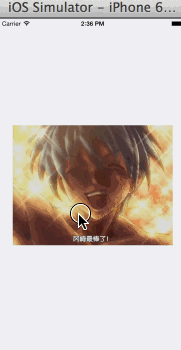# 矩阵变换

## 基向量

$\stackrel{\to }{a}=\left(x,0\right),\stackrel{\to }{b}=\left(0,y\right)，有\stackrel{\to }{v}=\stackrel{\to }{a}+\stackrel{\to }{b}$

$\stackrel{\to }{a}=x\stackrel{\to }{i},\stackrel{\to }{b}=y\stackrel{\to }{j}$

$\stackrel{\to }{v}=\stackrel{\to }{a}+\stackrel{\to }{b}=x\stackrel{\to }{i}+y\stackrel{\to }{j}$

$\stackrel{\to }{v}=n\stackrel{\to }{i}+m\stackrel{\to }{j}=\left(na,nb\right)+\left(mc,md\right)=\left(na+mc,nb+md\right)=\left(x,y\right)$

$\left\{\begin{array}{rl}x& =na+mc\\ y& =nb+md\end{array}$

$n=\frac{dx-cy}{ad-bc},m=\frac{ay-bx}{ad-bc}$

$\stackrel{\to }{v}=n\stackrel{\to }{i}+m\stackrel{\to }{j}=\frac{dx-cy}{ad-bc}\stackrel{\to }{i}+\frac{ay-bx}{ad-bc}\stackrel{\to }{j}$

## 线性变换

vector f(vector v) {
vector x = v进行某些计算后的结果
return x;
}

$\stackrel{\to }{x}=f\left(\stackrel{\to }{v}\right)$

ok，这“某些条件”是哪些条件啊？按照书上对线性的定义，函数需要满足：

$f\left(\stackrel{\to }{v}+\stackrel{\to }{u}\right)=f\left(\stackrel{\to }{v}\right)+f\left(\stackrel{\to }{u}\right)$

$f\left(c\stackrel{\to }{v}\right)=cf\left(\stackrel{\to }{v}\right)$

$\stackrel{\to }{i}=\left(1,0\right),\stackrel{\to }{j}=\left(0,1\right)$

$L\left(\stackrel{\to }{v}\right)=L\left(x\stackrel{\to }{i}+y\stackrel{\to }{j}\right)=L\left(x\stackrel{\to }{i}\right)+L\left(y\stackrel{\to }{j}\right)=xL\left(\stackrel{\to }{i}\right)+yL\left(\stackrel{\to }{j}\right)$

$L\left(\stackrel{\to }{v}\right)=xL\left(\stackrel{\to }{i}\right)+yL\left(\stackrel{\to }{j}\right)$

$\stackrel{\to }{v}=x\stackrel{\to }{i}+y\stackrel{\to }{j}$

$\stackrel{\to }{u}=L\left(\stackrel{\to }{v}\right),\stackrel{\to }{k}=L\left(\stackrel{\to }{i}\right),\stackrel{\to }{l}=L\left(\stackrel{\to }{j}\right)$

$\begin{array}{}\text{(1)}& \stackrel{\to }{u}=x\stackrel{\to }{k}+y\stackrel{\to }{l}\end{array}$

$\begin{array}{}\text{(2)}& \stackrel{\to }{v}=x\stackrel{\to }{i}+y\stackrel{\to }{j}\end{array}$

$\begin{array}{}\text{(3)}& L\left(\stackrel{\to }{v}\right)=x\stackrel{\to }{k}+y\stackrel{\to }{l}=\left(x,y\right)\left(\begin{array}{c}\stackrel{\to }{k}\\ \stackrel{\to }{l}\end{array}\right)\end{array}$

$L\left(\stackrel{\to }{v}\right)=x\stackrel{\to }{k}+y\stackrel{\to }{l}=\left(x,y\right)\left(\begin{array}{c}\stackrel{\to }{k}\\ \stackrel{\to }{l}\end{array}\right)=\left(x,y\right)\left(\begin{array}{cc}a& b\\ c& d\end{array}\right)=\left(x,y\right){A}_{t}=\stackrel{\to }{v}{A}_{t}$

$L\left(\stackrel{\to }{v}\right)=\stackrel{\to }{v}{A}_{t}，其中{A}_{t}=\left(\begin{array}{c}\stackrel{\to }{k}\\ \stackrel{\to }{l}\end{array}\right)=\left(\begin{array}{cc}a& b\\ c& d\end{array}\right)$

vector linear_transformation(vector v) {
// 找到该变换对应的矩阵
matrix At = (a,b,c,d);
// 输入向量左乘矩阵A
vector u = vAt;

return u;
}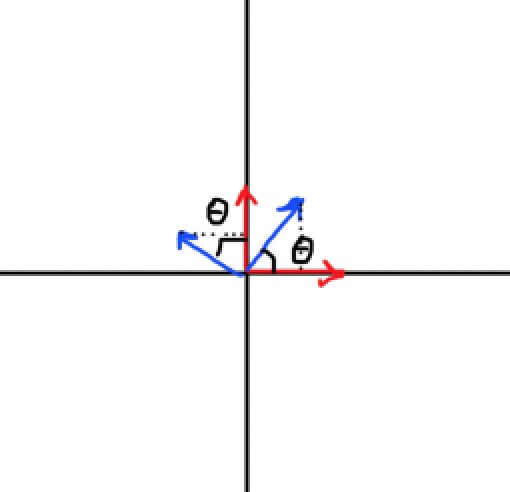$\stackrel{\to }{k}=\left(\mathrm{cos}\theta ,\mathrm{sin}\theta \right)，\stackrel{\to }{l}=\left(-\mathrm{sin}\theta ,\mathrm{cos}\theta \right)$

$A=\left(\begin{array}{cc}\mathrm{cos}\theta & \mathrm{sin}\theta \\ -\mathrm{sin}\theta & \mathrm{cos}\theta \end{array}\right)$

$\stackrel{\to }{u}=L\left(\stackrel{\to }{v}\right)=\stackrel{\to }{v}{A}_{t}=\left(x,y\right)\left(\begin{array}{cc}\mathrm{cos}\theta & \mathrm{sin}\theta \\ -\mathrm{sin}\theta & \mathrm{cos}\theta \end{array}\right)=\left(x\mathrm{cos}\theta -y\mathrm{sin}\theta ,x\mathrm{sin}\theta +y\mathrm{cos}\theta \right)$

## 线性变换的复合

$\stackrel{\to }{w}=\stackrel{\to }{u}{A}_{scale}=\left(\stackrel{\to }{v}{A}_{rotate}\right){A}_{scale}$

$\stackrel{\to }{w}=\left(\stackrel{\to }{v}{A}_{rotate}\right){A}_{scale}=\stackrel{\to }{v}\left({A}_{rotate}{A}_{scale}\right)=\stackrel{\to }{v}{A}_{t}$

${A}_{t}={A}_{rotate}{A}_{scale}$

# 齐次坐标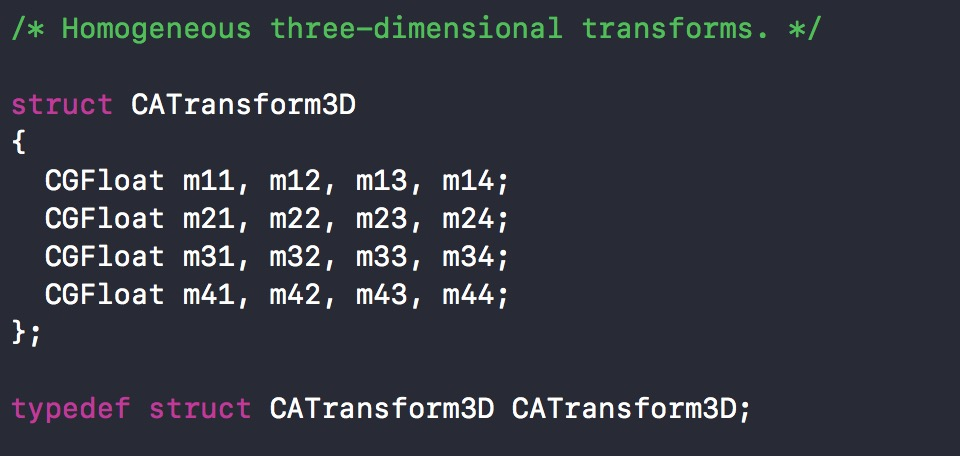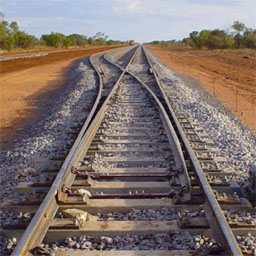## 齐次坐标下的点和向量的区别

$\begin{array}{}\text{(1)}& \stackrel{\to }{v}=x\stackrel{\to }{i}+y\stackrel{\to }{j}\end{array}$

$P-O=\stackrel{\to }{v}$

$\begin{array}{}\text{(2)}& P\left(x,y\right)=x\stackrel{\to }{i}+y\stackrel{\to }{j}+O\end{array}$

$\stackrel{\to }{v}=\left(x,y\right)\left(\begin{array}{c}\stackrel{\to }{i}\\ \stackrel{\to }{j}\end{array}\right)=\left(x,y,0\right)\left(\begin{array}{c}\stackrel{\to }{i}\\ \stackrel{\to }{j}\\ O\end{array}\right)$

$P=\left(x,y,1\right)\left(\begin{array}{c}\stackrel{\to }{i}\\ \stackrel{\to }{j}\\ O\end{array}\right)$

## 齐次坐标下的平移变换

$\left(x,y\right){A}_{t}=\left(x+a,y+b\right)$

$\left(x,y,1\right){A}_{t}=\left(x+a,y+b,1\right)$

${A}_{rotate}=\left(\begin{array}{ccc}cos& sin& 0\\ -sin& cos& 0\\ 0& 0& 1\end{array}\right),{A}_{scale}=\left(\begin{array}{ccc}sx& 0& 0\\ 0& sy& 0\\ 0& 0& 1\end{array}\right)$

$\left(x,y,0\right){A}_{t}=\left(x+a,y+b,0\right)$

$f\left(x,y\right)=\left(x+a,y+b\right)$

$f\left(U+V\right)=f\left({u}_{x}+{v}_{x},{u}_{y}+{v}_{y}\right)=\left({u}_{x}+{v}_{x}+a,{u}_{y}+{v}_{y}+b\right)$

$f\left(U\right)+f\left(V\right)=f\left({u}_{x},{u}_{y}\right)+f\left({v}_{x},{v}_{y}\right)\phantom{\rule{0ex}{0ex}}=\left({u}_{x}+a,{u}_{y}+b\right)+\left({v}_{x}+a,{v}_{y}+b\right)\phantom{\rule{0ex}{0ex}}=\left({u}_{x}+{v}_{x}+2a,{u}_{y}+{v}_{y}+2b\right)$

$f\left(U+V\right)\ne f\left(U\right)+f\left(V\right)$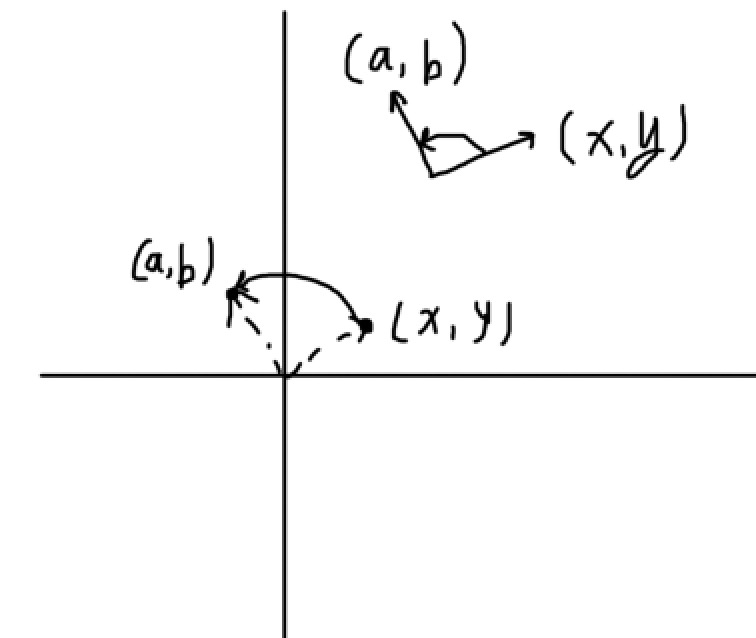## 齐次坐标下的平行线相交问题

$\left\{\begin{array}{r}Ax+By+C=0\\ Ax+By+D=0\end{array}$

$\left\{\begin{array}{r}A\frac{{x}^{\prime }}{k}+B\frac{{y}^{\prime }}{k}+C=0\\ A\frac{{x}^{\prime }}{k}+B\frac{{y}^{\prime }}{k}+D=0\end{array}$

$\left\{\begin{array}{r}A{x}^{\prime }+B{y}^{\prime }+Ck=0\\ A{x}^{\prime }+B{y}^{\prime }+Dk=0\end{array}$

# CATransform3D

1. 图像是由像素点构成的，要实现图像的各种变形变换，需要一个变换函数，将一个点作为输入参数，输出变换后的点。把构成该图像的所有像素点都拿去调用这个函数，就能实现图像的变形了；
2. 在线性代数中有一种变换叫线性变换，它接收一个向量作为输入参数，输出变形后的向量，比如一个逆时针旋转90°的线性变换，接收任何一个向量，输出的向量就是原向量逆时针旋转90°后的向量；
3. 线性变换的过程实际上就是输入向量乘以某个变换矩阵。我们需要实现的三种基本变换：平移、缩放、旋转中，缩放和旋转是线性变换（满足可加性和一阶齐次），由于矩阵乘法拥有结合律，所以多个线性变换可以通过它们的变换矩阵相乘复合成一个变换矩阵，比如我们可以用缩放矩阵乘以旋转矩阵，得到的结果就是一个描述先缩放再旋转的变换矩阵，输入向量乘以这个矩阵，输出的向量就是先缩放后旋转的结果；
4. 对于平移变换，为了让它也能通过矩阵乘法进行变换的复合，我们发现只有在齐次坐标下才能找到这样一个矩阵，而为了能让平移变换也加入旋转和缩放的复合运算中，旋转和缩放也应该在齐次坐标下来表示，这样它们才能相乘。

## 基于CATransform3D的变换矩阵

// 让一个layer在x轴方向拉伸2倍，在y轴方向拉伸3倍，在z轴方向拉伸1倍。
// 当然一般的layer是没有厚度的概念的，所以z轴的拉伸对layer而言就是没有意义的
layer.transform = CATransform3DMakeScale(2, 3, 1);

/* Returns a transform that scales by (sx, sy, sz)':
* t' = [sx 0 0 0; 0 sy 0 0; 0 0 sz 0; 0 0 0 1]. */

CA_EXTERN CATransform3D CATransform3DMakeScale (CGFloat sx, CGFloat sy,
CGFloat sz)
CA_AVAILABLE_STARTING (10.5, 2.0, 9.0, 2.0);

${t}^{\prime }=\left(\begin{array}{cccc}sx& 0& 0& 0\\ 0& sy& 0& 0\\ 0& 0& sz& 0\\ 0& 0& 0& 1\end{array}\right)$

$x轴的基向量\stackrel{\to }{i}=\left(1,0,0\right),y轴的基向量\stackrel{\to }{j}=\left(0,1,0\right),z轴的基向量\stackrel{\to }{k}=\left(0,0,1\right)$$x轴的基向量\vec{i} = (1,0,0),y轴的基向量\vec{j} = (0,1,0),z轴的基向量\vec{k} = (0,0,1)$

$\stackrel{\to }{si}=\left(sx,0,0\right),\stackrel{\to }{sj}=\left(0,sy,0\right),\stackrel{\to }{sk}=\left(0,0,sz\right)$

$t=\left(\begin{array}{ccc}sx& 0& 0\\ 0& sy& 0\\ 0& 0& sz\end{array}\right)$

/* Returns a transform that translates by '(tx, ty, tz)':
* t' =  [1 0 0 0; 0 1 0 0; 0 0 1 0; tx ty tz 1]. */

CA_EXTERN CATransform3D CATransform3DMakeTranslation (CGFloat tx,
CGFloat ty, CGFloat tz)
CA_AVAILABLE_STARTING (10.5, 2.0, 9.0, 2.0);

/* Returns a transform that scales by (sx, sy, sz)':
* t' = [sx 0 0 0; 0 sy 0 0; 0 0 sz 0; 0 0 0 1]. */

CA_EXTERN CATransform3D CATransform3DMakeScale (CGFloat sx, CGFloat sy,
CGFloat sz)
CA_AVAILABLE_STARTING (10.5, 2.0, 9.0, 2.0);

/* Returns a transform that rotates by 'angle' radians about the vector
* '(x, y, z)'. If the vector has length zero the identity transform is
* returned. */

CA_EXTERN CATransform3D CATransform3DMakeRotation (CGFloat angle, CGFloat x,
CGFloat y, CGFloat z)
CA_AVAILABLE_STARTING (10.5, 2.0, 9.0, 2.0);

/* Translate 't' by '(tx, ty, tz)' and return the result:
* t' = translate(tx, ty, tz) * t. */

CA_EXTERN CATransform3D CATransform3DTranslate (CATransform3D t, CGFloat tx,
CGFloat ty, CGFloat tz)
CA_AVAILABLE_STARTING (10.5, 2.0, 9.0, 2.0);

/* Scale 't' by '(sx, sy, sz)' and return the result:
* t' = scale(sx, sy, sz) * t. */

CA_EXTERN CATransform3D CATransform3DScale (CATransform3D t, CGFloat sx,
CGFloat sy, CGFloat sz)
CA_AVAILABLE_STARTING (10.5, 2.0, 9.0, 2.0);

/* Rotate 't' by 'angle' radians about the vector '(x, y, z)' and return
* the result. If the vector has zero length the behavior is undefined:
* t' = rotation(angle, x, y, z) * t. */

CA_EXTERN CATransform3D CATransform3DRotate (CATransform3D t, CGFloat angle,
CGFloat x, CGFloat y, CGFloat z)
CA_AVAILABLE_STARTING (10.5, 2.0, 9.0, 2.0);

/* Concatenate 'b' to 'a' and return the result: t' = a * b. */

CA_EXTERN CATransform3D CATransform3DConcat (CATransform3D a, CATransform3D b)
CA_AVAILABLE_STARTING (10.5, 2.0, 9.0, 2.0);


// 这是一个数学公式而不是赋值语句
CATransform3DScale(t,sx,sy,sz) = CATransform3DConcat(t,CATransform3DMakeScale(sx,sy,sz))


/* The identity transform: [1 0 0 0; 0 1 0 0; 0 0 1 0; 0 0 0 1]. */

CA_EXTERN const CATransform3D CATransform3DIdentity

## 3D旋转变换

/* Returns a transform that rotates by 'angle' radians about the vector
* '(x, y, z)'. If the vector has length zero the identity transform is
* returned. */

CA_EXTERN CATransform3D CATransform3DMakeRotation (CGFloat angle, CGFloat x,
CGFloat y, CGFloat z)
CA_AVAILABLE_STARTING (10.5, 2.0, 9.0, 2.0);

- (void)touchesEnded:(NSSet<UITouch *> *)touches withEvent:(UIEvent *)event
{
CALayer * layer = [CALayer layer];
layer.frame = CGRectMake(0, 0, 320, 240);
layer.position = self.view.center;
layer.contents = (__bridge id)[UIImage imageNamed:@"1.jpg"].CGImage;

// 为了让效果更直观，我们写个动画出来看，让layer绕着x轴旋转的动画
CABasicAnimation * animation = [CABasicAnimation animation];
animation.keyPath = @"transform";
animation.duration = 5;
animation.removedOnCompletion = NO;
animation.fillMode = kCAFillModeForwards;
// 绕x轴旋转π/4

CATransform3D transform = CATransform3DMakeRotation(M_PI/4, 1, 0, 0);

animation.toValue = [NSValue valueWithCATransform3D:transform];
}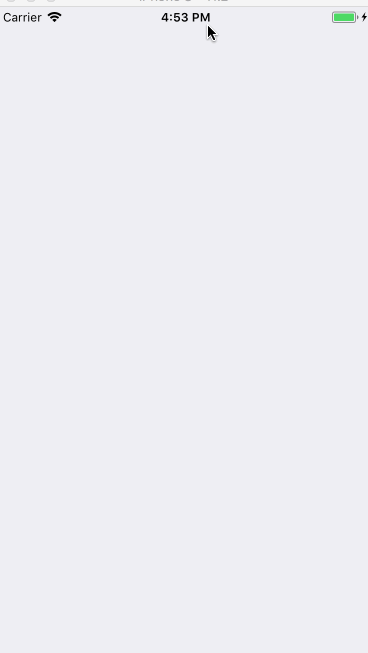## 带透视效果的CATransform3D旋转

- (void)touchesEnded:(NSSet<UITouch *> *)touches withEvent:(UIEvent *)event
{
CALayer * layer = [CALayer layer];
layer.frame = CGRectMake(0, 0, 320, 240);
layer.position = self.view.center;
layer.contents = (__bridge id)[UIImage imageNamed:@"1.jpg"].CGImage;

CABasicAnimation * animation = [CABasicAnimation animation];
animation.keyPath = @"transform";
animation.duration = 5;
animation.removedOnCompletion = NO;
animation.fillMode = kCAFillModeForwards;

// 设置透视变换矩阵
CATransform3D perspectiveTransform = CATransform3DIdentity;
perspectiveTransform.m34 = -1.f/700;
// 将透视变换复合到旋转变换中
// 绕x轴旋转π/4
CATransform3D transform = CATransform3DRotate(perspectiveTransform, M_PI/4, 1, 0, 0);

animation.toValue = [NSValue valueWithCATransform3D:transform];
}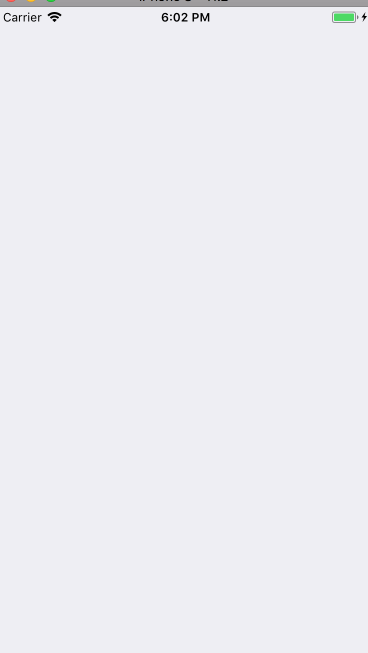# 基于Pan手势的3D旋转控制

## 总体实现思路

1. 这是一个3D旋转效果；
2. 这个3D旋转效果是由pan手势控制的；
3. 旋转的方向就是手指移动的方向。

CATransform3D CATransform3DRotate (CATransform3D t, CGFloat angle,
CGFloat x, CGFloat y, CGFloat z)

## 通过向量计算当前手指的移动方向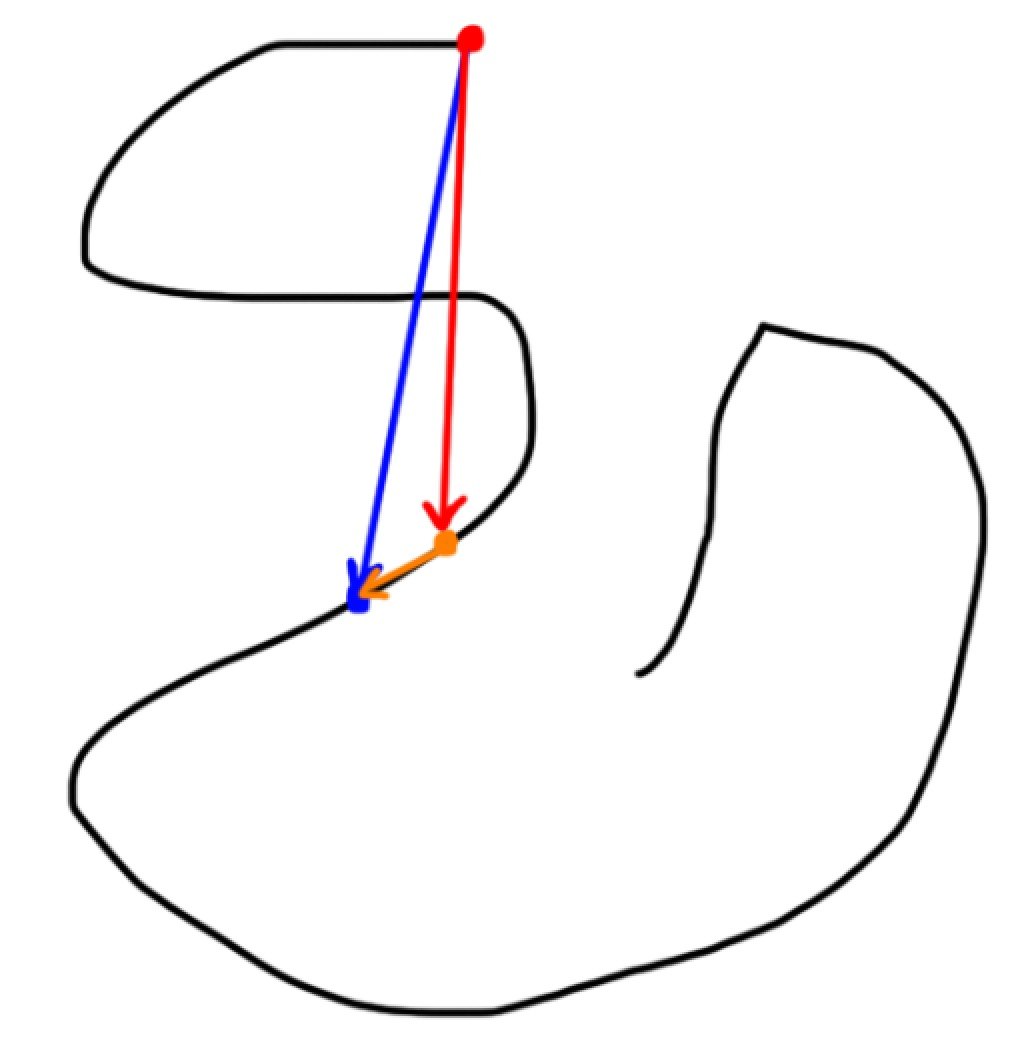$\stackrel{\to }{{v}_{红}}+\stackrel{\to }{{v}_{橙}}=\stackrel{\to }{{v}_{蓝}}=>\stackrel{\to }{{v}_{橙}}=\stackrel{\to }{{v}_{蓝}}-\stackrel{\to }{{v}_{红}}$

- (void)onPanGesture:(UIPanGestureRecognizer *)sender
{
if (sender.state == UIGestureRecognizerStateBegan) {

} else if (sender.state == UIGestureRecognizerStateChanged) {

// 获取当前手指的位移（translation）
CGPoint panTranslation = [sender translationInView:sender.view];
// 我们要的向量是手指上次所在的点到这次所在的点连成的一个向量，这是你这次手指滑动的方向，传给transform3DRotate函数的向量是垂直于这个向量的向量。而我们已知的只有这个transition，也就是手指最开始的点到手指当前点连成的一个向量（也就是手指的位移，只考虑起始点和结束点）。
// 画出图来就发现，我们要的向量就是当前向量-上一次手指的位移向量（向量减法）

// 通过这个位移生成一个向量，这就是我们当前的位移向量。
DHVector * vector = [[DHVector alloc] initWithCoordinateExpression:panTranslation];

// 用当前的位移向量-上次的位移向量得到我们手指的位移偏移量
DHVector * translateVector = [DHVector aVector:vector substractedByOtherVector:[self lastTranslation]];

// 把这个向量保存起来，下次调用这个方法的时候需要拿到这次的向量，用来做减法
// 下次再调用这个方法的时候的lastTranslation就是这次的位移向量，所以用这次的位移向量覆盖掉lastTranslation（用这次的位移向量给lastTranslation赋值）
[self setLastTranslation:vector];

// 随便计算一下单位旋转角度，也就是每次调用这个方法的时候应该旋转多少度（线性插值）

// 生成旋转向量，也就是要传给CATransform3DRotate函数的向量，它通过translateVector顺时针旋转90度（PI/2）得到
DHVector * rotateVector = [DHVector vectorWithVector:translateVector];

// 把旋转向量传给函数
self.layer.transform = CATransform3DRotate(self.layer.transform, radian, rotateVector.coordinateExpression.x,  rotateVector.coordinateExpression.y, 0);

} else if (sender.state == UIGestureRecognizerStateCancelled || sender.state == UIGestureRecognizerStateEnded) {

}
}


// 手指的最大位移量和当手指达到最大位移量时对应的旋转角度，用来插值计算每次手指移动应该旋转多少度
static const CGFloat maxTranslate_ = 400.f;
static const CGFloat maxRotateRadian_   =   M_PI * 2;

@interface ViewController ()

@property (nonatomic, strong) UIView * transformView;
@property (nonatomic, strong) DHVector * lastTranslation;
@property (nonatomic, assign) CGFloat transformUnit;

@end

@implementation ViewController

self.view.backgroundColor = [UIColor groupTableViewBackgroundColor];

self.transformUnit = 1.5;

}

- (void)setTransform3DWithPanTranslation:(CGPoint)translation
{
// 我们要的向量是手指上次所在的点到这次所在的点连成的一个向量，这是你这次手指滑动的方向，传给transform3DRotate函数的向量是垂直于这个向量的向量。而我们已知的只有这个transition，也就是手指最开始的点到手指当前点连成的一个向量（也就是手指的位移，只考虑起始点和结束点）。
// 画出图来就发现，我们要的向量就是当前向量-上一次手指的位移向量（向量减法）

// 通过这个位移生成一个向量。
DHVector * vector = [[DHVector alloc] initWithCoordinateExpression:translation];

// 用当前的位移向量-上次的位移向量得到我们手指的位移偏移量
DHVector * translateVector = [DHVector aVector:vector substractedByOtherVector:[self lastTranslation]];

// 把这个向量保存起来，下次调用这个方法的时候需要拿到这次的向量，用来做减法
[self setLastTranslation:vector];

// 随便计算一下单位旋转角度，也就是每次调用这个方法的时候应该旋转多少度

// 生成旋转向量，也就是要传给CATransform3DRotate函数的向量，它通过translateVector顺时针旋转90度（PI/2）得到
DHVector * rotateVector = [DHVector vectorWithVector:translateVector];

// 把旋转向量传给函数
self.transformView.layer.transform = CATransform3DRotate(self.transformView.layer.transform, radian, rotateVector.coordinateExpression.x,  rotateVector.coordinateExpression.y, 0);
}

#pragma mark - callback
- (void)onPanGesture:(UIPanGestureRecognizer *)sender
{
if (sender.state == UIGestureRecognizerStateBegan) {

} else if (sender.state == UIGestureRecognizerStateChanged) {

[self setTransform3DWithPanTranslation:[sender translationInView:sender.view]];

} else if (sender.state == UIGestureRecognizerStateCancelled || sender.state == UIGestureRecognizerStateEnded) {

}
}

#pragma mark - getter

- (UIView *)transformView
{
if (!_transformView) {
_transformView = ({

UIView * view = [[UIView alloc] initWithFrame:CGRectMake(0, 0, 320, 240)];
view.transformUnit = 1.5;
view.center = self.view.center;
view.backgroundColor = [UIColor blueColor];
view.layer.contents = (__bridge id)[UIImage imageNamed:@"1.jpg"].CGImage;
[view prepareForTransform3D];
view;

});

}
return _transformView;
}

@end


# 总结

CoreAnimation专题的最后一篇终于结束了，整个实践篇的目的在于让大家通过我们原理篇和技巧篇的内容来解决需求中可能遇到的各种各样的动画难题，所以我在实践篇的写作中大量提及思考的过程，阅读起来可能会比较难啃，比较干涩（毕竟都是干货呢），但是我的想法是让大家读完实践篇后不仅能实现实践篇里面那么几个效果，还能拥有动画实现的基本思路（套路，即各种分解动画的思维方式），结合我们的技巧篇的各种工具，能够见招拆招，遇到什么都不怕，这样才是内力的修炼，而不是只会几个固定的招数。内力修炼的过程是比较漫长而痛苦的，我也是一步一步一个坑一个坑走过来的，希望大家都能有所收获吧！

3d ios 翻转动画 相关内容

2016-04-06 15:57:37 boring_cat 阅读数 311
• ###### 老镇讲堂-Swift动画编程指南

掌握Swift动画编程

25854 人正在学习 去看看 曾伟（老镇）

①还未旋转之前②旋转中③旋转后#import <UIKit/UIKit.h>

@interface TransView : UIView

/** 二维动画旋转*/
-(void)transition2DwithRotation:(CGFloat) transform Duration:(CGFloat)time;

/** 三维动画旋转*/

@end

#import "TransView.h"

@implementation TransView

-(instancetype)initWithFrame:(CGRect)frame{

if (self = [super initWithFrame:frame]) {
self.frame = frame;
}

return self;

}

/** 二维动画旋转*/
-(void)transition2DwithRotation:(CGFloat) transform Duration:(CGFloat)time{

//让view旋转
[UIView animateWithDuration:time animations:^{

self.transform = CGAffineTransformMakeRotation(transform);

} completion:^(BOOL finished) {

}];

}

/** 三维动画旋转*/

//方法一
[UIView animateWithDuration:time animations:^{

self.layer.transform = transform3D;

} completion:^(BOOL finished) {

if (finishImage) {

self.backgroundColor = [UIColor colorWithPatternImage:finishImage];
}

[UIView animateWithDuration:time animations:^{
self.layer.transform = CATransform3DMakeRotation(M_PI, 0, 1, 0);
}];

}];

//方法二
//    [UIView transitionWithView:self duration:time options:UIViewAnimationOptionTransitionFlipFromLeft animations:^{
//        if (finishImage) {
//            //默认图片
//            self.backgroundColor = [UIColor colorWithPatternImage:[UIImage imageNamed:@"user_default"]];
//        }
//    } completion:^(BOOL finished) {
//
//        if (finishImage) {
//            //旋转后的图片
//            self.backgroundColor = [UIColor colorWithPatternImage:finishImage];
//        }
//     });
//
//
//    }];
}

CATransform3DMakeRotation(M_PI, 0, 1, 0);

M_PI 3.14159265358979323846264338327950288 /* pi */
M_PI_2 1.57079632679489661923132169163975144 /* pi/2 */
M_PI_4 0.785398163397448309615660845819875721 /* pi/4 */
M_1_PI 0.318309886183790671537767526745028724 /* 1/pi */
M_2_PI 0.636619772367581343075535053490057448 /* 2/pi */

view.transform 是二维旋转
view.layer.transform 图层可三维旋转

3d ios 翻转动画 相关内容

2014-08-08 15:43:27 piaol 阅读数 138
• ###### 老镇讲堂-Swift动画编程指南

掌握Swift动画编程

25854 人正在学习 去看看 曾伟（老镇）

#define kDegreesToRadian(x) (M_PI * (x) / 180.0)

{

self.title = @"测试动画";

self.view.backgroundColor = [UIColorlightGrayColor];

myTest1 = [[UILabelalloc]initWithFrame:CGRectMake(10, 100, 60, 40)];

myTest1.backgroundColor = [UIColorblueColor];

myTest1.textAlignment = NSTextAlignmentCenter;

myTest1.text = @"张明炜";

myTest1.textColor = [UIColorwhiteColor];

//闪烁效果。

///移动的动画。

//    [myTest1.layer addAnimation:[self moveX:1.0f X:[NSNumber numberWithFloat:200.0f]] forKey:nil];

//缩放效果。

//    [myTest1.layer addAnimation:[self scale:[NSNumber numberWithFloat:1.0f] orgin:[NSNumber numberWithFloat:3.0f] durTimes:2.0f Rep:MAXFLOAT] forKey:nil];

//组合动画。

//    NSArray *myArray = [NSArray arrayWithObjects:[self opacityForever_Animation:0.5],[self moveX:1.0f X:[NSNumber numberWithFloat:200.0f]],[self scale:[NSNumber numberWithFloat:1.0f] orgin:[NSNumber numberWithFloat:3.0f] durTimes:2.0f Rep:MAXFLOAT], nil];

//    [myTest1.layer addAnimation:[self groupAnimation:myArray durTimes:3.0f Rep:MAXFLOAT] forKey:nil];

//路径动画。

//    CGMutablePathRef myPah = CGPathCreateMutable();

//    CGPathMoveToPoint(myPah, nil,30, 77);

//    CGPathAddCurveToPoint(myPah, nil, 50, 50, 60, 200, 200, 200);//这里的是控制点。

//    [myTest1.layer addAnimation:[self keyframeAnimation:myPah durTimes:5 Rep:MAXFLOAT] forKey:nil];

//旋转动画。

}

#pragma mark === 永久闪烁的动画 ======

-(CABasicACnimation *)opacityForever_Animation:(float)time

{

CABasicAnimation *animation = [CABasicAnimationanimationWithKeyPath:@"opacity"];//必须写opacity才行。

animation.fromValue = [NSNumbernumberWithFloat:1.0f];

animation.toValue = [NSNumbernumberWithFloat:0.0f];//这是透明度。

animation.autoreverses = YES;

animation.duration = time;

animation.repeatCount = MAXFLOAT;

animation.removedOnCompletion = NO;

animation.fillMode = kCAFillModeForwards;

animation.timingFunction=[CAMediaTimingFunctionfunctionWithName:kCAMediaTimingFunctionEaseIn];///没有的话是均匀的动画。

return animation;

}

#pragma mark =====横向、纵向移动===========

-(CABasicAnimation *)moveX:(float)time X:(NSNumber *)x

{

CABasicAnimation *animation = [CABasicAnimationanimationWithKeyPath:@"transform.translation.x"];///.y的话就向下移动。

animation.toValue = x;

animation.duration = time;

animation.removedOnCompletion = NO;//yes的话，又返回原位置了。

animation.repeatCount = MAXFLOAT;

animation.fillMode = kCAFillModeForwards;

return animation;

}

#pragma mark =====缩放-=============

-(CABasicAnimation *)scale:(NSNumber *)Multiple orgin:(NSNumber *)orginMultiple durTimes:(float)time Rep:(float)repertTimes

{

CABasicAnimation *animation = [CABasicAnimationanimationWithKeyPath:@"transform.scale"];

animation.fromValue = Multiple;

animation.toValue = orginMultiple;

animation.autoreverses = YES;

animation.repeatCount = repertTimes;

animation.duration = time;//不设置时候的话，有一个默认的缩放时间.

animation.removedOnCompletion = NO;

animation.fillMode = kCAFillModeForwards;

return  animation;

}

#pragma mark =====组合动画-=============

-(CAAnimationGroup *)groupAnimation:(NSArray *)animationAry durTimes:(float)time Rep:(float)repeatTimes

{

CAAnimationGroup *animation = [CAAnimationGroupanimation];

animation.animations = animationAry;

animation.duration = time;

animation.removedOnCompletion = NO;

animation.repeatCount = repeatTimes;

animation.fillMode = kCAFillModeForwards;

return animation;

}

#pragma mark =====路径动画-=============

-(CAKeyframeAnimation *)keyframeAnimation:(CGMutablePathRef)path durTimes:(float)time Rep:(float)repeatTimes

{

CAKeyframeAnimation *animation = [CAKeyframeAnimationanimationWithKeyPath:@"position"];

animation.path = path;

animation.removedOnCompletion = NO;

animation.fillMode = kCAFillModeForwards;

animation.timingFunction = [CAMediaTimingFunctionfunctionWithName:kCAMediaTimingFunctionEaseIn];

animation.autoreverses = NO;

animation.duration = time;

animation.repeatCount = repeatTimes;

return animation;

}

#pragma mark ====旋转动画======

-(CABasicAnimation *)rotation:(float)dur degree:(float)degree direction:(int)direction repeatCount:(int)repeatCount

{

CATransform3D rotationTransform = CATransform3DMakeRotation(degree, 0, 0, direction);

CABasicAnimation *animation = [CABasicAnimationanimationWithKeyPath:@"transform"];

animation.toValue = [NSValue valueWithCATransform3D:rotationTransform];

animation.duration  =  dur;

animation.autoreverses = NO;

animation.cumulative = NO;

animation.fillMode = kCAFillModeForwards;

animation.repeatCount = repeatCount;

animation.delegate = self;

return animation;

}

3d ios 翻转动画 相关内容